Test: Network Theorems (A.C.) - 1

# Test: Network Theorems (A.C.) - 1

Test Description

## 10 Questions MCQ Test GATE Electrical Engineering (EE) 2023 Mock Test Series | Test: Network Theorems (A.C.) - 1

Test: Network Theorems (A.C.) - 1 for Electrical Engineering (EE) 2023 is part of GATE Electrical Engineering (EE) 2023 Mock Test Series preparation. The Test: Network Theorems (A.C.) - 1 questions and answers have been prepared according to the Electrical Engineering (EE) exam syllabus.The Test: Network Theorems (A.C.) - 1 MCQs are made for Electrical Engineering (EE) 2023 Exam. Find important definitions, questions, notes, meanings, examples, exercises, MCQs and online tests for Test: Network Theorems (A.C.) - 1 below.
Solutions of Test: Network Theorems (A.C.) - 1 questions in English are available as part of our GATE Electrical Engineering (EE) 2023 Mock Test Series for Electrical Engineering (EE) & Test: Network Theorems (A.C.) - 1 solutions in Hindi for GATE Electrical Engineering (EE) 2023 Mock Test Series course. Download more important topics, notes, lectures and mock test series for Electrical Engineering (EE) Exam by signing up for free. Attempt Test: Network Theorems (A.C.) - 1 | 10 questions in 30 minutes | Mock test for Electrical Engineering (EE) preparation | Free important questions MCQ to study GATE Electrical Engineering (EE) 2023 Mock Test Series for Electrical Engineering (EE) Exam | Download free PDF with solutions
 1 Crore+ students have signed up on EduRev. Have you?
Test: Network Theorems (A.C.) - 1 - Question 1

### Determine VTH for the circuit external to RL in the given figure.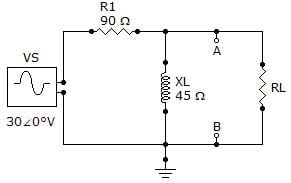Test: Network Theorems (A.C.) - 1 - Question 2

### Referring to the given circuit, how much power, in watts, is delivered to the speaker at the determined frequency if VS = 4.5 VRMS? Block Diagram: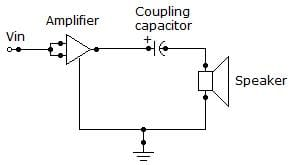Circuit Diagram: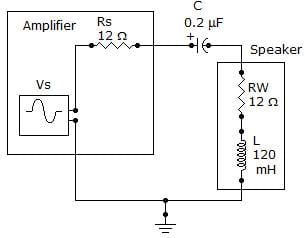Test: Network Theorems (A.C.) - 1 - Question 3

### If two currents are in the same direction at any instant of time in a given branch of a circuit, the net current at that instant

Test: Network Theorems (A.C.) - 1 - Question 4

Norton's theorem gives

Test: Network Theorems (A.C.) - 1 - Question 5

Referring to the given circuit, determine ZTH as seen by RL.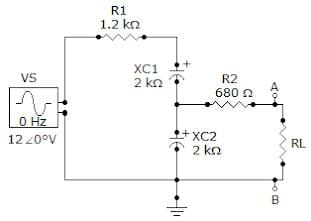Test: Network Theorems (A.C.) - 1 - Question 6

Determine the frequency at which the maximum power is transferred from the amplifier to the speaker in the given figure.

Block Diagram:Circuit Diagram:Test: Network Theorems (A.C.) - 1 - Question 7

Referring to the given circuit, what is ZTH if R1 is changed to 220 Ω?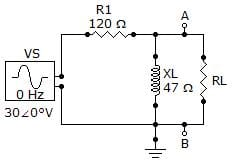Test: Network Theorems (A.C.) - 1 - Question 8

Referring to the given circuit, what is VTH if VS = 12 ∠0° V?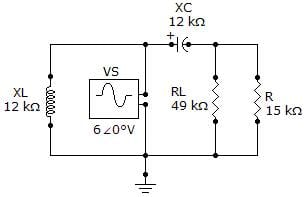Test: Network Theorems (A.C.) - 1 - Question 9

The two basic components of a Thevenin equivalent ac circuit are

Test: Network Theorems (A.C.) - 1 - Question 10

In Superposition theorem, while considering a source, all other current sources are?

Detailed Solution for Test: Network Theorems (A.C.) - 1 - Question 10

In Superposition theorem, while considering a source, all other current sources are open circuited. Superposition theorem is not valid for power responses. It is applicable only for computing voltage and current responses.

## GATE Electrical Engineering (EE) 2023 Mock Test Series

22 docs|274 tests
Information about Test: Network Theorems (A.C.) - 1 Page
In this test you can find the Exam questions for Test: Network Theorems (A.C.) - 1 solved & explained in the simplest way possible. Besides giving Questions and answers for Test: Network Theorems (A.C.) - 1, EduRev gives you an ample number of Online tests for practice

## GATE Electrical Engineering (EE) 2023 Mock Test Series

22 docs|274 tests(Scan QR code)